# Data Structures 101: implement hash tables in JavaScript

Sep 08, 2020 - 11 min readJerry Ejonavi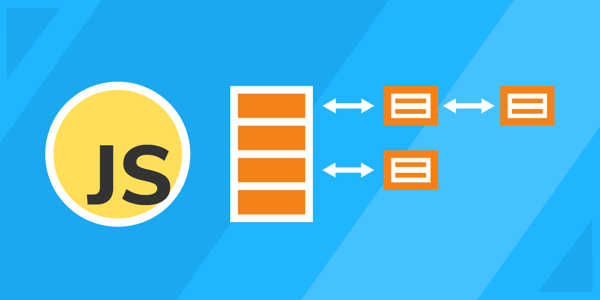In computer programming, data structures are used to organize data and apply algorithms (or commands) to code. Having a good understanding of data structures and algorithms is useful for efficient problem-solving and essential to cracking coding interviews.

We will continue with our data structures series with one of the top data structures, the Hash Table. We’ll learn what they are, what they are used for, and how to implement them in JavaScript.

Today, we will cover:

## What is a hash table?

A hash table (often called a hash map) is a data structure that maps keys to values. Hash tables combine lookup, insert, and delete operations in an efficient way. The key is sent to a hash function that performs arithmetic operations on it. The result (called the hash value or hash) is an index of the key-value pair.

Think of this like a signature on a block of data that allows us to search in constant time. A hash table operates like a dictionary that we can map to get from the hash to the desired data.

This data structure is widely used in many kinds of computer software, particularly for associative arrays, database indexing, caches, and sets. Usually, this operation returns the same hash for a given key.

The performance of a hash table depends on three fundamental factors: hash function, size of the hash table, and collision handling method.

Hash tables are made up of two parts:

• Object: An object with the table where the data is stored. The array holds all the key-value entries in the table. The size of the array should be set according to the amount of data expected.
• Hash function (or mapping function): This function determines the index of our key-value pair. It should be a one-way function and produce the a different hash for each key.

Note: In JavaScript, hash tables are generally implemented using arrays as they provide access to elements in constant time.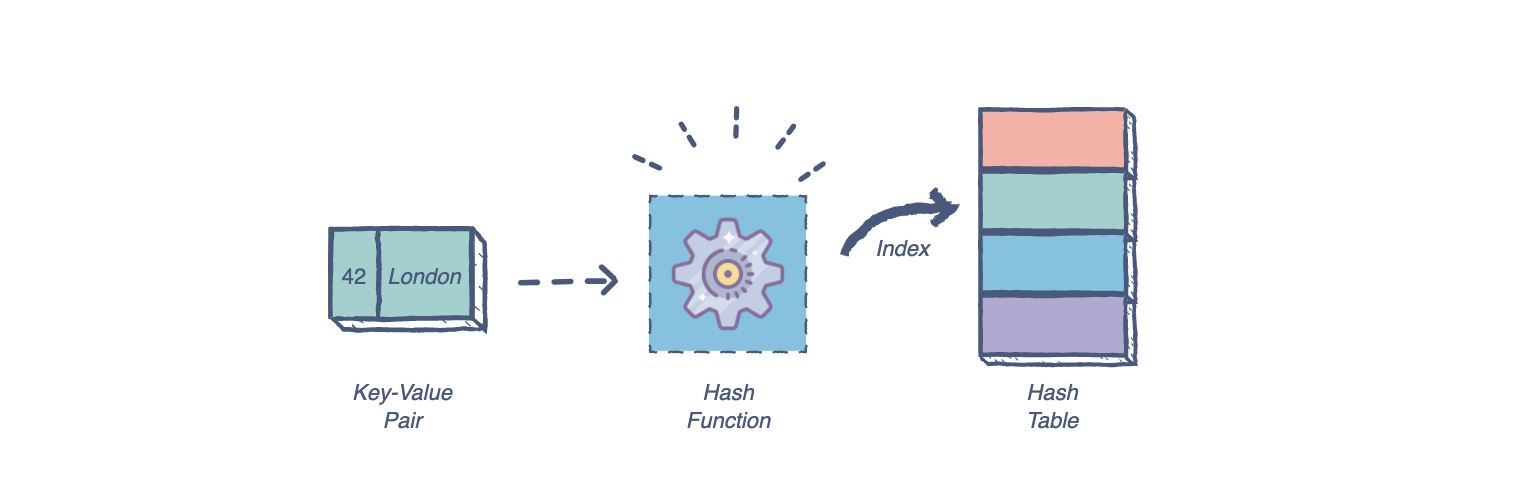### Uses of hash tables

Hash tables provide access to elements in constant time, so they are highly recommended for algorithms that prioritize search and data retrieval operations. Hashing is ideal for large amounts of data, as they take a constant amount of time to perform insertion, deletion, and search.

In terms of time complexity, the operation is $0(1)$. On average, a hash table lookup is more efficient than other table lookup data structures. Some common uses of hash tables are:

• Database indexing
• Caches
• Unique data representation
• Lookup in an unsorted array
• Lookup in sorted array using binary search

### Hash tables vs. trees

Hashing and trees perform similar jobs, but various factors in your program determine when to use one over the other.

Trees are more useful when an application needs to order data in a specific sequence. Hash tables are the smarter choice for randomly sorted data due to its key-value pair organization.

Hash tables can perform in constant time, while trees usually work in $O(log n)$. In the worst-case scenario, the performance of hash tables can be as low as $O(n)$. An AVL tree, however, would maintain $O(log n)$ in the worst case.

An efficient hash table requires a hash function to distribute keys. A tree is simpler, since it accesses extra space only when needed and does not require a hash function.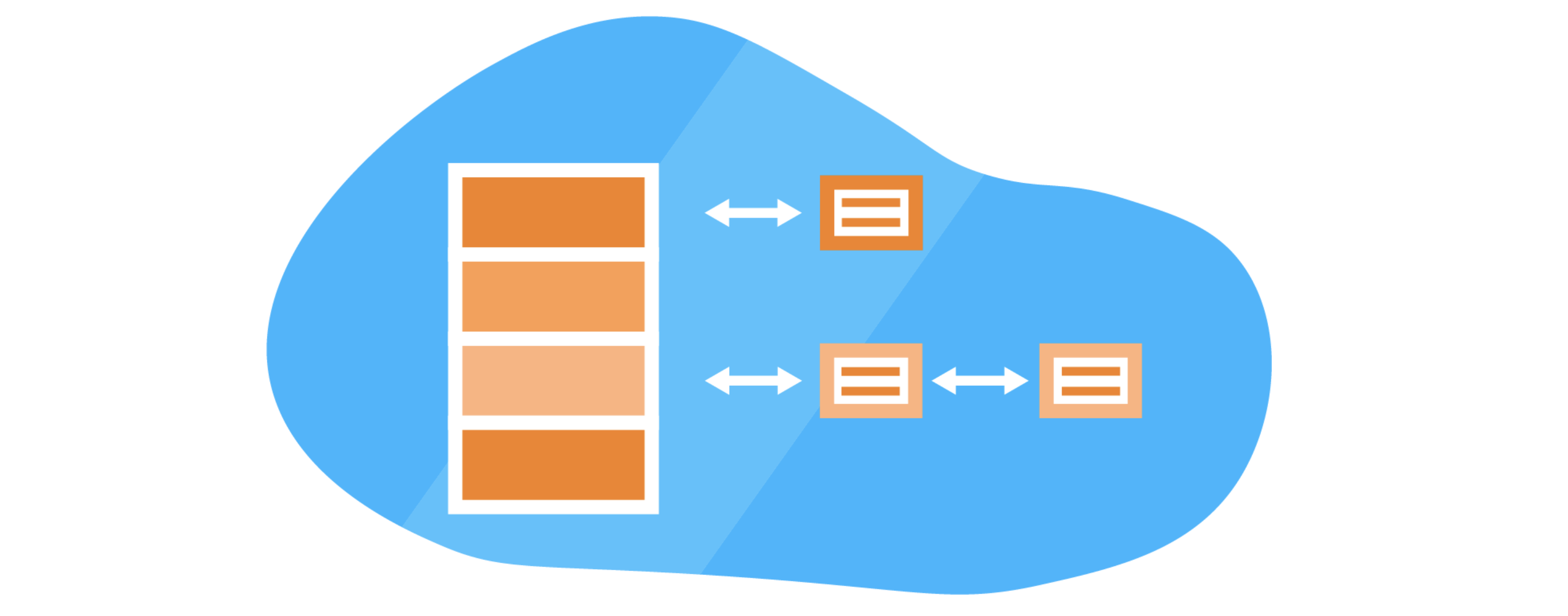## What is a hash function?

A hash function is a method or function that takes an item’s key as an input, assigns a specific index to that key and returns the index whenever the key is looked up. This operation usually returns the same hash for a given key. A good hash function should be efficient to compute and uniformly distribute keys.

Hash functions help to limit the range of the keys to the boundaries of the array, so we need a function that converts a large key into a smaller key. This is the job of the hash function.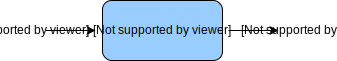### Common hash functions

There are many kinds of hash functions that have different uses. Let’s take a look at some of the most common hash functions used in modern programming.

Arithmetic Modular: In this approach, we take the modular of the key with the list/array size: index=key MOD tableSize. So, the index will always stay between 0 and tableSize - 1.

Truncation: Here, we select a part of the key as the index rather than the whole key. We can use a mod function for this operation, although it does not need to be based on the array size

Folding: This approach involves dividing the key into small chunks and applying a different arithmetic strategy at each chunk.

## Hash table collisions

Sometimes, a hash function can generate the same index for more than one key. This scenario is referred to as a hash collision. Collisions are a problem because every slot in a hash table is supposed to store a single element.

Hash collisions are usually handled using four common strategies.

1. Linear probing: Linear probing works by skipping over an index that is already filled. It could be achieved by adding an offset value to an already computed index. If that index is also filled, add it again and so on.

One drawback of using this strategy is that if you don’t pick an offset wisely, you can jump back to where you started and miss out on so many possible positions in the array.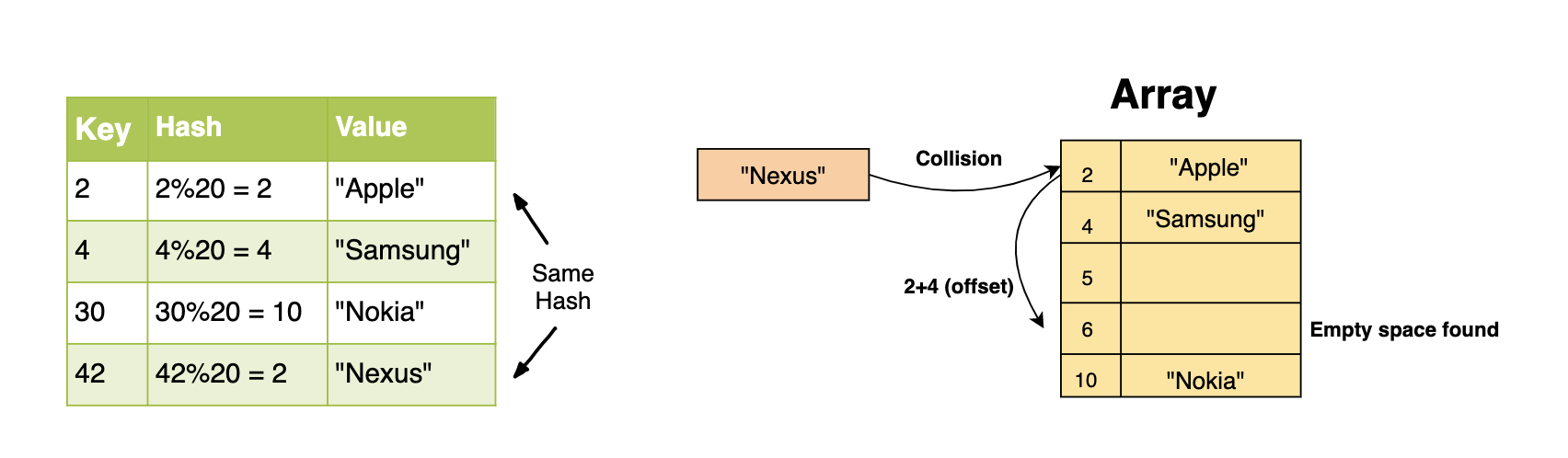1. Chaining: In the chaining strategy, each slot of our hash table holds a pointer to another data structure such as a linked list or a tree. Every entry at that index will be inserted into the linked list for that index.

As you can see, chaining allows us to hash multiple key-value pairs at the same index in constant time (insert at head for linked lists). This strategy greatly increases performance, but it is costly in terms of space.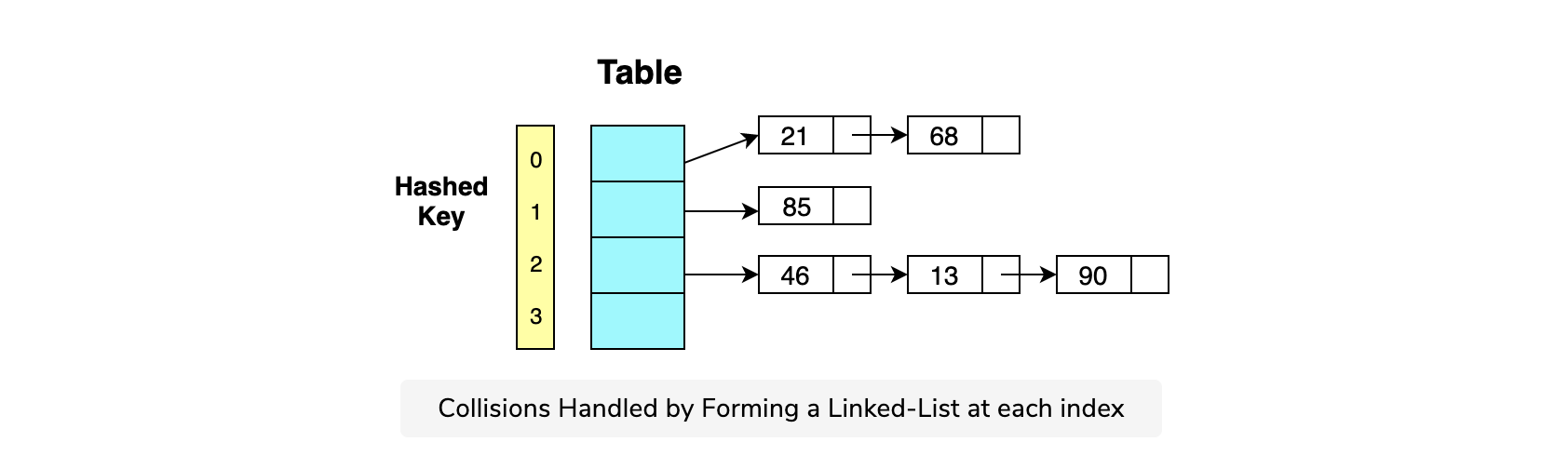1. Resizing the Array or List: Another way to reduce collisions is to resize the list or array. We can set a threshold and once it is crossed, we can create a new table which is double the size of the original. All we have to do then is to copy the elements from the previous table.

Resizing the list or array significantly reduces collisions, but the function itself is costly. Therefore, we need to be careful about the threshold we set. A typical convention is to set the threshold at 0.6, which means that when 60% of the table is filled, the resize operation needs to take place.

2. Double hashing: In double hashing, there are two hash functions. The second hash function is used to provide an offset value in case the first function causes a collision. Double hashing can find the next free slot faster than a linear probing approach. This is useful for applications with a smaller hash table.

The following function is an example of double hashing:

(firstHash(key) + i * secondHash(key)) % tableSize


### Master data structures for coding interviews.

Learn data structures and algorithms in JavaScript without scrubbing through videos or documentation. Educative’s text-based courses are easy to skim and feature live coding environments, making learning quick and efficient.

Data Structures for Coding Interviews in JavaScript

## How to implement a hash table

To implement a hash table using JavaScript, we will do three things: create a hash table class, add a hash function, and implement a method for adding key/value pairs to our table.

First, let’s create the HashTable class.

class HashTable {
constructor() {
this.values = {};
this.length =  0;
this.size =  0;
}
}

The constructor contains an object in which we’re going to store the values, the length of the values, and the entire size of the hash table: meaning how many buckets the hash table contains. We will be storing our data in these buckets.

Next, we have to implement a simple hashing function.

calculateHash(key) {
return key.toString().length % this.size;
}

This function takes the provided key and returns a hash that’s calculated using an arithmetic modulus.

Finally, we need a method to insert key/value pairs. Take a look at the code and see this in action:

add(key, value) {
const hash = this.calculateHash(key);
if (!this.values.hasOwnProperty(hash)) {
this.values[hash] = {};
}
if (!this.values[hash].hasOwnProperty(key)) {
this.length++;
}
this.values[hash][key] = value;
}

The first thing we do here is calculate a hash for our key. If the hash does not already exist, we save it to our object store. The next check we perform is on the hash. If it doesn’t have a key saved, we save the key and value and increment the size of our hash table.

Searching in a hash table goes very fast. Unlike with an array where we have to go through all of the elements until we reach the item we need, with a hash table we simply get the index. I’ll add the complete code for our hash table implementation below.

class HashTable {
constructor() {
this.values = {};
this.length =  0;
this.size =  0;
}

calculateHash(key) {
return key.toString().length % this.size;
}

const hash = this.calculateHash(key);
If (!this.values.hasOwnProperty(hash)) {
this.values[hash] = {};
}
if (!this.values[hash].hasOwnProperty(key)) {
this.length++;
}
this.values[hash][key] = value;
}

search(key) {
const hash = this.calculateHash(key);
if (this.values.hasOwnProperty(hash) && this.values[hash].hasOwnProperty(key)) {
return this.values[hash][key];
} else {
return null;
}
}
}

//create object of type hash table
const ht = new HashTable();
//add data to the hash table ht

//search
console.log(ht.search("Italy"));

That completes our basic JavaScript Hash Table implementation. Note that we used an Object to represent our hash table. Objects in JavaScript are actually implemented using hash tables themselves! Many programming languages also provide support for hash tables either as built-in associative arrays or as standard library modules.

### Implementation with Bucket Chaining

Now, let’s see another implementation using bucket chaining. The chaining strategy along with the resize operation to avoid collisions in the table.

All elements with the same hash key will be stored in an array at that index. In data structures, these arrays are called buckets. The size of the hash table is set as $n*m$ where $n$ is the number of keys it can hold, and $m$ is the number of slots each bucket contains.We will start by building a simple HashEntry class. As discussed earlier, a typical hash entry consists of three data members: the key, the value and the reference to a new entry.

class HashEntry{
constructor(key, data){
this.key = key;
// data to be stored
this.value = data;
// reference to new entry
this.next = null;
}
}

let entry = new HashEntry(3, "Educative");
console.log (String(entry.key) + ", " + entry.value);

Now, we’ll create the HashTable class which is a collection of HashEntry objects. We will keep track of the number of slots in the table and the current size of the hash table. These variables​ will come in handy when we need to resize the table.

class HashTable{
//Constructor
constructor(){
//Size of the HashTable
this.slots = 10;
//Current entries in the table
//Used while resizing the table when half of the table gets filled
this.size = 0;
//Array of HashEntry objects (by deafult all None)
this.bucket = [];
for (var i=0; i<this.slots; i++){
this.bucket[i]=null;
}
}
//Helper Functions
get_size(){
return this.size;
}
isEmpty(){
return this.get_size() == 0;
}
}

let ht = new HashTable();
console.log(ht.isEmpty());

The last thing we need is a hash function. We tried out some different approaches in the previous lessons. For our implementation, we will simply take the modular of the key with the total size of the hash table (slots).

//Hash Function
getIndex(key){
let index = key % this.slots;
return index;
}

There we go! The next steps would be to implement the operations of search, insertion, and deletion one by one.

## Wrapping up and interview questions

Congratulations on making it to the end of this article! I hope this has been a good starting point as you continue your journey to learn more about hash tables and data structures generally.

The next thing to learn are common interview questions for hash tables, so you can see how to implement operations and map a hash table. Some of these include:

• Implement insertion, delete, and search
• Check if arrays are disjointed
• Find symmetric pair in an array
• Find two pairs such that $a+b = c+d$
• Find two numbers that add up to “value”
• Remove duplicates from a Linked List using hash tables
• How to insert a new value to a hash key that is already occupied
• and more

If you’re ready to move onto real-world problems, check out the course Data Structures for Coding Interviews in JavaScript. It contains a detailed review of all the common data structures and provides implementation level details in JavaScript to help you become well equipped with all the different data structures.

By the end, you’ll have all the hands-on practice you need to ace your next coding interview.

Happy learning!